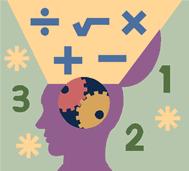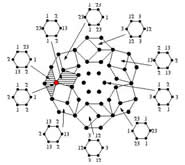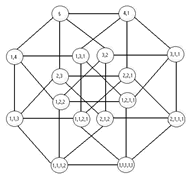# Hire/Pay a math expert to do your math assignments, homework, or online class

Our experts can give you full step-by-step solutions to any mathematics question. If you have an assignment to do to or simply want a solution to a single question your own math genius can either help you do the job or simply do it for you. If you have a difficult assignment coming up or simply want to work with your own personal math genius to practice for an exam, rest assured, you are in safe hands.

The following is a broad description of just a few of everyday areas of mathematics in which we have served hundreds of customers:

Calculus
Our experts understand and give complete answers to questions on limits, derivatives, integrals and infinite series which often become very difficult for even the best of students. As one of the primary branches of mathematics, calculus has quite a few applications in science and engineering. Students that are taking mathematics as a part of their scientific coursework can greatly benefit from seeing complete solutions to their problems that can help them understand how a particular problem is supposed to be handled. Calculus is often considered harder than Algebra alone but these two topics are often covered simultaneously at university level courses. In this manner, it constitutes a major part of modern university education. Calculus is one of the hardest sub areas of mathematics since it involves a large number of rules and theorems related to various geometric functions. Commonly, even students in the liberal arts would be required to take at least one calculus course. Such a course may be taught to a large class by only one teacher which leaves little time for explanations and step by step procedural explanation of problems. We believe that this can be a major cause for the problems faced with calculus with university students. The broad focus of most university level courses is on integration and differentiation related problems therefore we seek out qualified experts to retain on our staff who can easily help with even the most difficult integration/differentiation related questions.Algebra
Algebra is also one of the primary fields in mathematics. Basic algebra is often included as a part of the curriculum in secondary education as well as in high school classes and the purpose for studying it is to get a deeper understanding of mathematical functions as they relate to variables, polynomials as well as factors and roots. From the secondary school level to the graduate level, algebra requires careful explanation and crystal clear understanding which can easily be obtained with our perfect solutions. At the college and university level, algebra becomes much broader and in addition to working with numbers, algebraic equations can include operations with symbols, variables, and sets. While the basics of algebra might be easy to understand for some students the more difficult areas such groups, rings and algebraic field equations are much harder to understand without accurate examples and completed solutions.Statistics
As a science, statistics is used in many different areas such as business, medicine, psychology as well as in mathematics. This particular field is directly connected with the collection, interpretation as well as the explanation of seemingly random data. We use statistics everyday when we make informed decisions about the chances of risks and rewards of our actions. Statistical methods are useful in understanding and generating patterns that help clear away randomness or uncertainty in scientific experiments. In higher level classes there is also the presence of mathematical statistics, which is concerned with the theoretical basis of statistics. The application of statistics as well as the theory behind statistics can be understood easily with our worked solutions that explain how the answer is arrived at.Discrete/Finite Mathematics
Highly important for computer science majors, discrete mathematics (which is also called finite mathematics) is the study of mathematical structures which are judged to need or not need continuity. Nearly every object studied in this field is a countable set of integers, graphs or even the words in a formal language. The application of the topic to computer science is obvious when we understand how computers define objects and properties of objects in ways which are similar to the equations in discrete mathematics. The subject is also applicable in some business courses but the primary connection of discrete mathematics remains with computers and programming languages. We have expert problem solvers for discrete mathematics from the top universities around the world working for us. They can certainly help and explain to you the process of handling even the most difficult questions.Combinatorics
At advanced levels of study, discrete mathematics may be studied under the topic of Combinatorics where several different fields of mathematics such as algebra, probability theory and even geometry may be combined together. The union of diverse mathematical fields can be difficult for many students to comprehend until they see how easy it is to actually solve problems that may seem daunting at first. The application of this field comes across in applied subjects such as computer science as well as statistical physics. Combinatorics is about problem solving and theory building, though it has been used to develop powerful theoretical methods, it remains difficult to understand and proper guidance is an essential requirement for all students.#### Math homework help

Here are some other math fields for which you can hire our services
Optimization Problems
Logic and Set Theory
Matrix Theory and Linear
Ring and Group Theory
Real and Complex Analysis

Your personal math genius can help you in all of the fields mentioned above and many others. There is little that we can not handle!